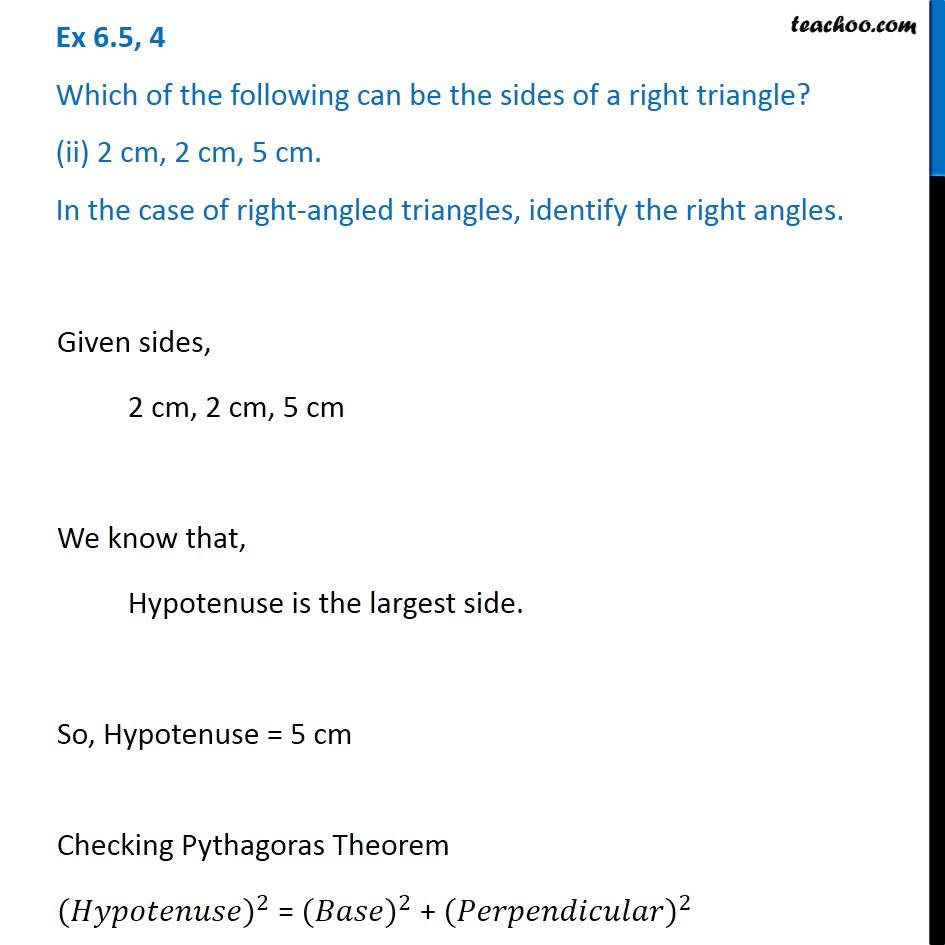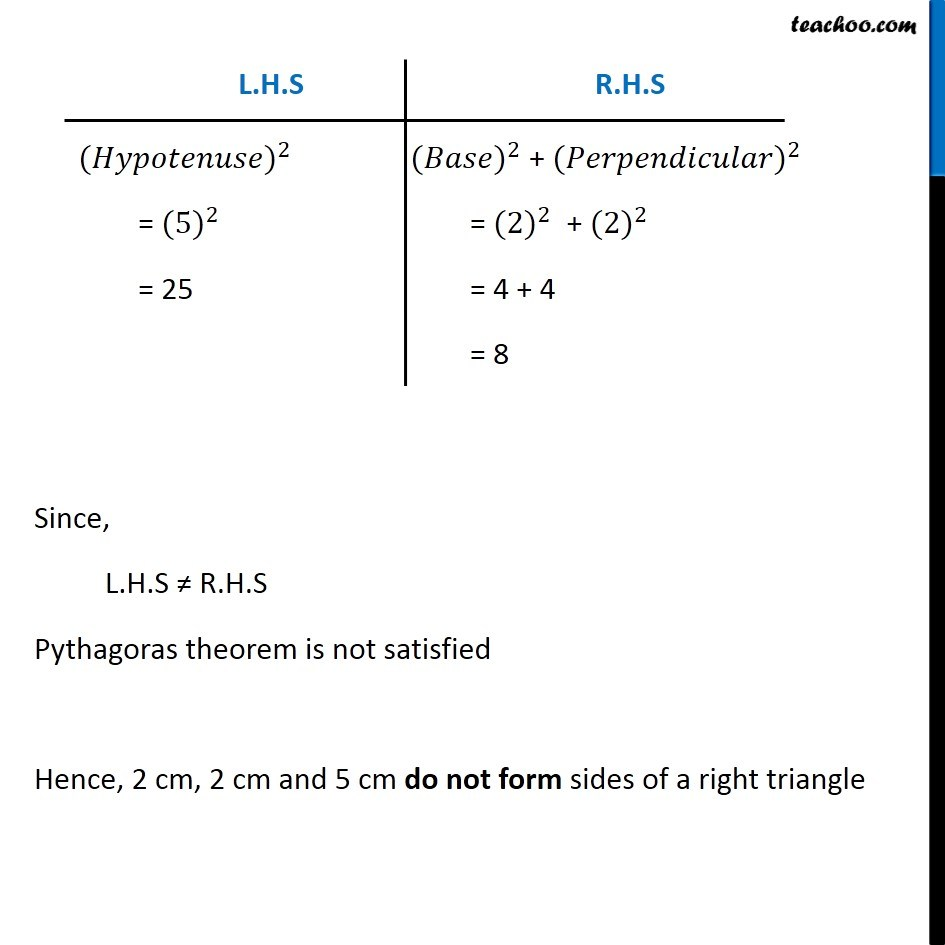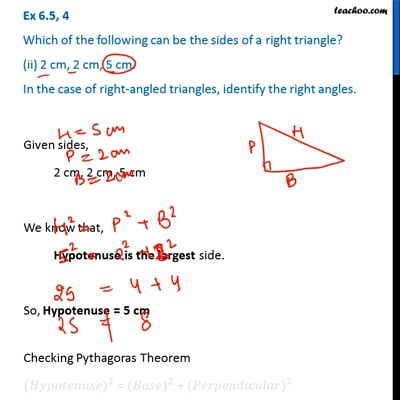Ex 6.5

Chapter 6 Class 7 Triangle and its Properties
Serial order wiseThis video is only available for Teachoo black users

Learn in your speed, with individual attention - Teachoo Maths 1-on-1 Class

### Transcript

Ex 6.5, 4 Which of the following can be the sides of a right triangle? (ii) 2 cm, 2 cm, 5 cm. In the case of right-angled triangles, identify the right angles. Given sides, 2 cm, 2 cm, 5 cm We know that, Hypotenuse is the largest side. So, Hypotenuse = 5 cm Checking Pythagoras Theorem 〖(𝐻𝑦𝑝𝑜𝑡𝑒𝑛𝑢𝑠𝑒)〗^2 = 〖(𝐵𝑎𝑠𝑒)〗^2 + 〖(𝑃𝑒𝑟𝑝𝑒𝑛𝑑𝑖𝑐𝑢𝑙𝑎𝑟)〗^2 〖(𝐻𝑦𝑝𝑜𝑡𝑒𝑛𝑢𝑠𝑒)〗^2 = 〖(5)〗^2 = 25 〖(𝐵𝑎𝑠𝑒)〗^2 + 〖(𝑃𝑒𝑟𝑝𝑒𝑛𝑑𝑖𝑐𝑢𝑙𝑎𝑟)〗^2 = 〖(2)〗^2 + 〖(2)〗^2 = 4 + 4 = 8 Since, L.H.S ≠ R.H.S Pythagoras theorem is not satisfied Hence, 2 cm, 2 cm and 5 cm do not form sides of a right triangle